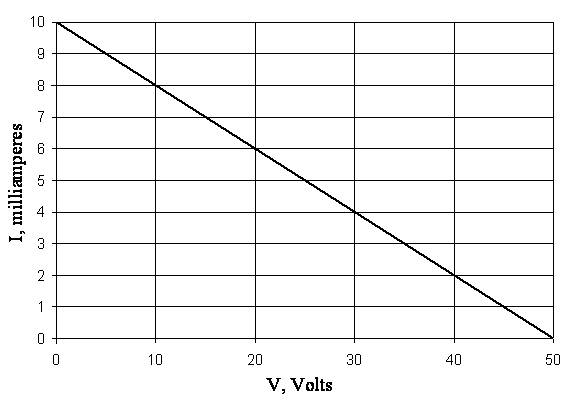# Homework Given January 16, 2007

## Due January23, 2007

These aren't homework problems per se; the Exercises are intended as a study aid for those reading the chapter.  These are selected to make sure that the intended points stick with the student.

## Live Links to the Exercises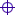Exercise 1.1Exercise 1.5Exercise 1.12

## Exercise 1.1

Plot the voltage-current curve of resistors of 1 k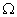, 5 k, and 20 k,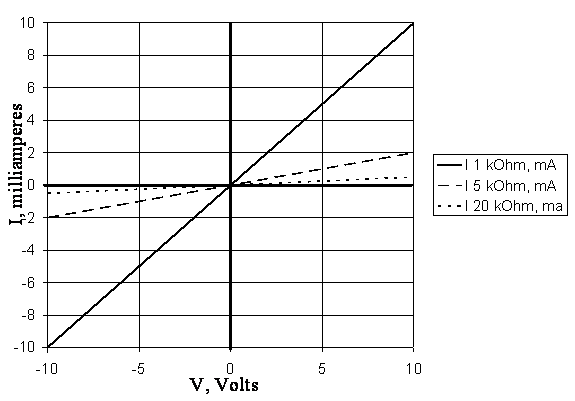## Exercise 1.5

Find the value of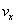in Figure 1.5 if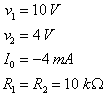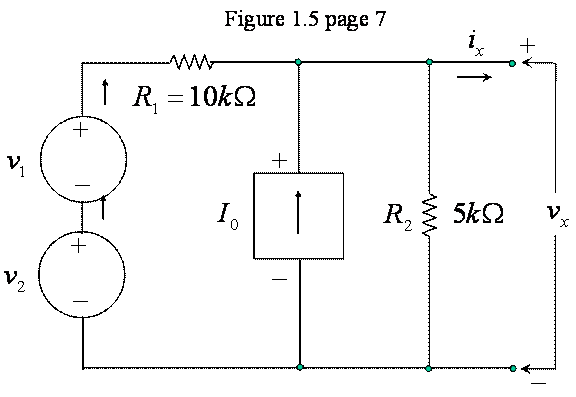It's tempting to use a Norton equivalent of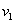,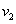, and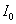, because that would result in two current sources in parallel across two resistors in parallel, a very simple circuit to solve.  However, the section is on superposition, so we will solve it by superposition.

The component offromis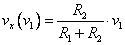and the componentfromis identical,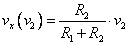.

The component offromis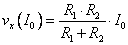.

The final value ofis the sum of the components from,, and,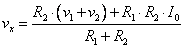.

For the values given in the problem statement,is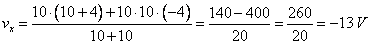.

## Exercise 1.12

Plot the v-I equation of the circuit of Figure 1.11 if the value of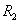is changed to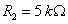.

The figure is part of Example 1.3, which is a superposition example.  To follow the intent of the exercise, we will solve it by superposition.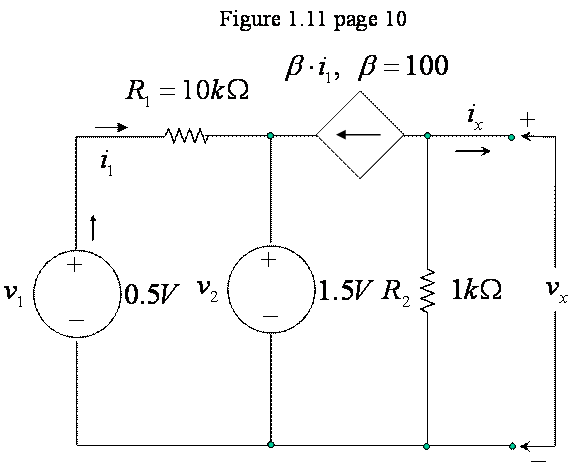Note that the zig-zag transistor model geometry of Figure 1.11 has been changed here to the more familiar ladder configuration that we use for circuit analysis.  To plot the v-I characteristic ofand, we need to find the open circuit voltage and short circuit current.  In class, I solved this circuit directly instead of using superposition as in Example 1.3, which is the context for the figure.  Here, we use superposition.

The component of the open circuit voltagedue tois found by finding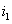and noting that the controlled current source andform a Norton equivalent across the terminals whichis measured.  This we have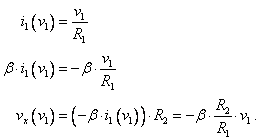The short circuit current is simply the controlled current source current, negated because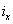is positive out the terminal, opposite the direction of the arrow in the controlled current source,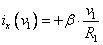.

The voltage sourcedrives current through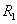opposite than that ofbut its effect on the controlled current source is otherwise identical, so we have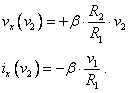By superposition, the open circuit voltage is the sum of the two voltages due toand, and the short circuit current is the sum of the currents due toand,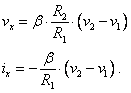For the values given in the problem,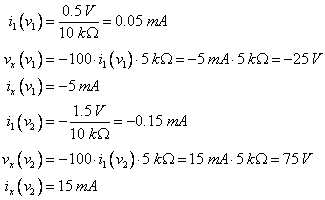and, finally, the total open circuit voltage and short circuit current are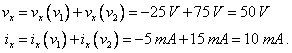We plot the v-i curve from the open circuit voltage, when current is zero, and the short circuit current, when the voltage is zero, as a straight line between these points as below.